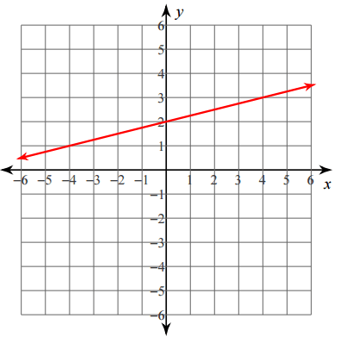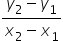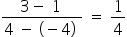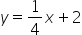Mathematics
Easy

Question

# Find the equation of the line that matches the graph.## y = 2xy = 1/4 x + 2y = 1x + 4y = -2xHint:

## The correct answer is: y = 1/4 x + 2

### From the graph, the y-intercept is 2.step1 :  taking ( 4 , 3 )  and ( -4 , 1 ) pointsslope of the line is (m) ==Substitute the values of m and b in slope-intercept form.     y = mx + cSlope of line is =### Related Questions to study#### With Turito Foundation.#### Get an Expert Advice From Turito.